# Protocols, Lesson 2: Binary and the Internet Protocol

## To understand the Internet Protocol, you need to understand Binary. Learn it here.

To understand the Internet Protocol, we need to learn and understand Binary. An important part of IP is subnetting, which can only be explained and understood when an IP address is converted to Binary. A lot of people are not aware that computers do not understand words, pictures and sounds when we interact with them by playing a game, reading or drawing something on the screen. The truth is that all computers can understand is zeros and ones. What we see on the screen is just an interpretation of what the computer understands, so the information displayed is useful and meaningful to us.

### Binary: Bits and bytes

To put it as simply as possible, a Bit is the smallest unit/value of Binary notation. The same way we say 1 cent is the smallest amount of money you can have, a Bit is the same thing but in Binary.

A Bit can have only one value, either a one or a zero. So if I gave you a value of zero (0) then you would say that is one Bit. If I gave you two of them (00), you would say that's two Bits.

Now, if you had eight zeros or ones together, as in 0110 1010 (I put a space in between to make it easier for the eyes), you would say that's 8 Bits or one Byte. Yes, that is correct; eight Bits are equal to one Byte. It doesn't matter if they are all ones or zeros or a mixture of the two.

The picture below gives you some examples: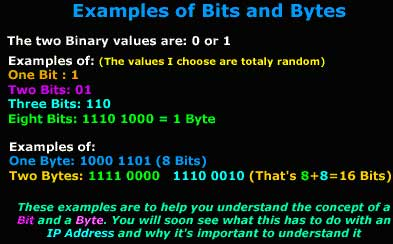To sum this all up, 1024 Bytes equal 1 Kbytes (Kilobyte). Why 1024 and not 1000? Well it's because of the way Binary works. If you did the math, you would find the above correct.

Everyone who uses the Internet would have, at one stage or another, come across the "Byte" or "Bit" term. This most frequently happens when you're downloading; you get the speed indication in bytes or Kbytes per second. We are going to see exactly what a Bit, Byte and Kbyte is, so you understand the terms.

### So, what's binary got to do with IP?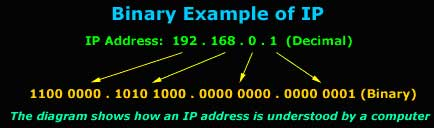The above example shows an IP address in decimal notation, which we understand more easily. This IP address (192.168.0.1) is then converted to Binary, which is what the computer understands. You can see how big the number gets. It's easier for us to remember four different numbers than 32 zeros or ones.

Now, keeping in mind what we said earlier about Bits and Bytes, have you ever heard or read people saying that an IP address is a 32 Bit address? It is, and you can now see why: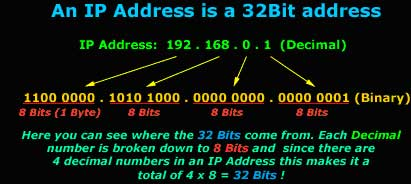So to sum up all the above, we now know what Binary notation is, what a Bit, Byte and Kbyte is and how Binary relates to an IP address, which is usually represented in its decimal notation.

Well, just as I explained in the introduction, computers display the zeros and ones in a way that makes the information useful to us. IP works a bit like this as well, where 98% of the time we see it in a decimal notation, but the computer understands it in binary. The picture below gives you an example of how a computer understands an IP address:

### Understanding the conversion between decimal and binary

The conversion is not that hard once you grasp the concept. The picture below shows an IP address that we are going to convert to Binary. Keep in mind that the method I'm going to show you is the same for all conversions. We are now going to convert the first octet in the IP address 192.168.0.1 to Binary/ In other words, we take the "192" and convert it to Binary. We are not going to have to do any difficult calculations, just simple additions: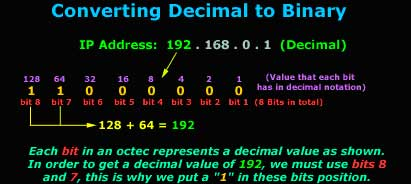If you have read and understood the first section of this page, you should know that we need eight bits to create one octet or, if you like, the 192 number. Each bit takes a certain value which never changes, and this value is shown in purple, right above the bit. We then select the bits we need in such a way that the sum of all selected bits gives us the decimal number we need.

If you wanted to explain the conversion in mathematical terms, you would say that each bit is a power of 2 (2^), for example, bit 8 is actually '2^7' = 128 in decimal, bit 7 is '2^6 = 64 in decimal, bit 6 is '2^5' = 32 in decimal, bit 5 is '2^4' = 16 in decimal, bit 4 is '2^3' = 8 in decimal, bit 3 is '2^2' = 4 in decimal, bit 2 is '2^1' = 2 in decimal, and bit 1 is '2^0' = 1 in decimal.

Note: When calculating the decimal value of an octet (192 in the example above), the Bit numbers do NOT represent the power of two value we must use in order to get the decimal value. This means that Bit 1 does NOT translate to 2^1=1 in decimal.

In our example, we used the 192. As you saw, we needed bits 8 and 7 and this gave us the Binary number of 11000000, which is 192 in decimal. You must remember that the values of each bit never change. For example, bit 8 always has a decimal value of 128, whereas bit 1 always takes the value of 1. Using this method, you will find it easy to convert decimal to Binary without the need for complex mathematical calculations.

So let's have a look at the next octet, which is the decimal number 168: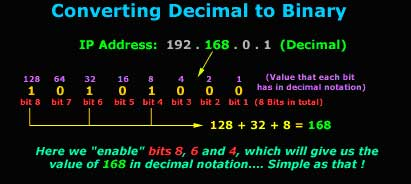Here again you can see that we needed to choose bits 8, 6 and 4 (in other words put a "1" in the bit's position) in order to get a decimal value of 168. So the Binary value of 10101000 is equal to the decimal value of 168.

Let's now look at all 4 octets of our IP address, in Binary: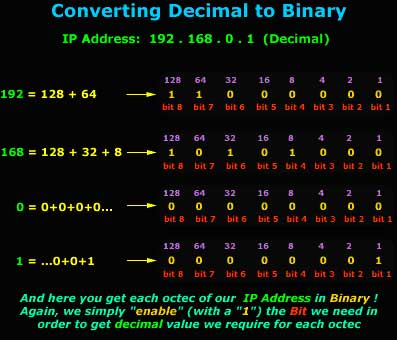No matter which way you convert, from Decimal to Binary or Binary to Decimal, the same method is used. If you understood the above, you should be able to convert any Binary or Decimal number.

That just about does it for this section; you're now ready for the next section!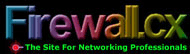Click over to Firewall.cx for more articles like this one. You don't have to register or jump through any hoops. All you do is get the networking information you want. Copyright 2004 Firewall.cx.
Now we're going to look at how the conversion works between decimal and binary. This is an important step, because you'll probably find yourself in need of such a conversion when dealing with complex subnets.
This was last published in October 2004

## SearchUnifiedCommunications

• ### Microsoft's Nuance buy enriches call center, security products

Analysts expect Microsoft to integrate Nuance voice technology with a wide range of Microsoft products, including Teams and ...

• ### Microsoft update of Teams Rooms for Android falls short

Microsoft has yet to add to Teams Rooms on Android critical features available on the Windows version. Missing features include ...

• ### 5 steps to managing team collaboration apps

Team collaboration apps offer measurable productivity and cost benefits. Follow these five steps to address technical, security ...

## SearchMobileComputing

• ### Chip shortage driving up PC prices, wait times

Businesses pay more for PCs and find it difficult to replace aging ones as the ongoing chip shortage leaves computer makers ...

• ### AR use cases gain ground due to COVID-19, maturing tech

AR technology is growing beyond technical instruction, helped by work-from-home mandates and maturing technology.

• ### Samsung introduces its cheapest A Series 5G phone yet

The Korean smartphone maker announced five additions to its midtier Galaxy A Series, including the A02s, A12, and three 5G phones...

## SearchDataCenter

• ### Programmable processor technology for next-gen data centers

The right processing technology can benefit your data center. Learn about advancements in CPU technologies, recent vendor ...

• ### Data processing units accelerate infrastructure performance

DPUs often run on networking packets to move information in the data center, instead of supporting processing workflows. Get an ...

• ### New Intel Ice Lake processors boost performance, security

Intel launches third-generation Xeon Scalable processors that bolster security, accelerate common data center workloads by 46% on...

## SearchITChannel

• ### IT industry's lack of diversity remains key issue in 2021

The first quarter of 2021 saw almost no changes in the composition of IT leadership teams. Here's how channel partners can change...

• ### How MSPs can build a post-merger integration team

Combining two companies is a complex undertaking, spanning employees, operations and line-of-business applications. An ...

• ### 3 basic cybersecurity measures to protect MSP businesses

Numerous MSP-targeted cyberattacks have driven home the need for improved internal security practices. Here's how MSPs can secure...

Close